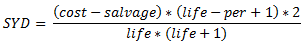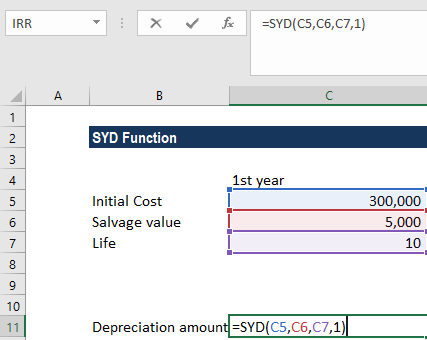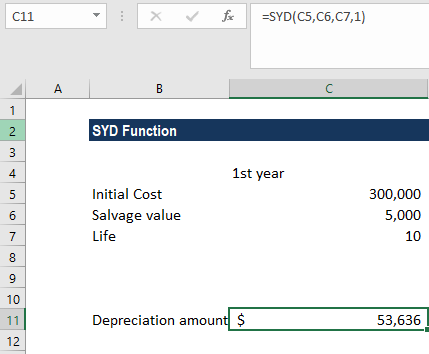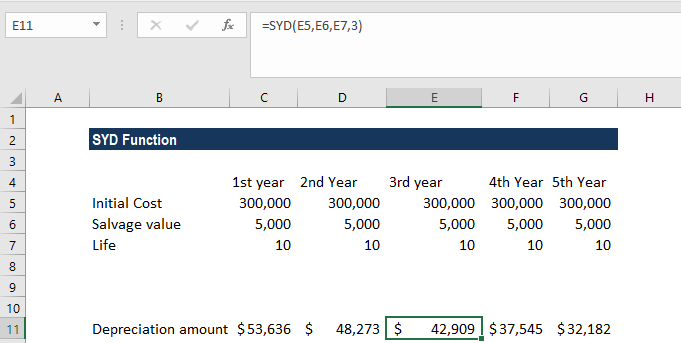# SYD Function

Calculates the sum-of-years' digits depreciation of an asset

## What is the SYD Function (Sum of Years Digits)?

The SYD Function is an Excel Financial function. SYD is short for sum of years digits. This function helps in calculating the depreciation of an asset, specifically the sum-of-years’ digits depreciation for a specified period in the lifetime of an asset.

Sum-of-years’ digits depreciation is a popular method in calculating the accelerated depreciation of an asset. The formula is as follows:Where:

• Cost is the initial cost of the asset (at start of period 1)
• Salvage is the final value of the asset at the end of its lifetime
• Life is the number of periods over which the depreciation occurs
• Per is the period for which the depreciation is being calculated

The SYD function is helpful to a financial analyst when building financial models or creating a fixed asset depreciation schedule for analysis.

### Sum of Years Digits (SYD) Formula

=SYD(cost, salvage, life, per)

The function uses the following arguments:

1. Cost (required argument) – The initial cost of the asset.
2. Salvage (required argument) – This is the value of the asset at the end of the depreciation. It can be zero. It is also known as the salvage value.
3. Life (required argument) – This is the useful life of the asset or the number of periods for which the asset will be depreciated.
4. Per (required argument) – The period for which we want to calculate the depreciation.

### How to use the SYD Function in Excel?

To understand the uses of the SYD function, let’s consider an example:

#### Example

Suppose we want to calculate the depreciation for an asset with an initial cost of \$300,000 and a salvage value of \$5,000 after 10 years.

We need to calculate the depreciation over five years. The formula for calculating the depreciation amount will be:We get the result below:For the subsequent years, we will change the per argument.

So for the second year, the formula would be =SYD(D5,D6,D7,2). For the third year, the formula would be =SYD(E5,E6,E7,3).

The result we get is shown below:### Things to remember about the SYD Function

1. We need to provide the arguments life and per in the same units of time: days, months, or years.
2. #VALUE! error – Occurs when the given arguments are non-numeric.
3. #NUM! error – Occurs when:
• The salvage argument provided is less than zero.
• The life or per argument given is less than or equal to zero.
• The per argument provided is greater than the life argument provided in the formula.

Thanks for reading CFI’s guide to the Excel SYD function. By taking the time to learn and master these functions, you’ll significantly speed up your financial analysis. To learn more, check out these additional CFI resources:

• Excel Functions for Finance